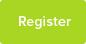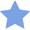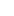# Tutor Hunt Questions

11g of ethyl ethanoate were mixed with 18cm^3 of 1.00moldm^-3 HCl(aq) in a flask and allowed to stand at a constant temperature until equilibrium was reached.

CH3CO2C2H5(l) + H2O(l) <=> (equilibrium) CH3CO2H(l) + C2H5OH(l)

Ethyl ethanoate + water <=> ethanoic acid + ethanol

When titrated with 1.00moldm^-3 NaOH(aq), 106cm^3 were required for neautralisation. Assume that 18cm^3 of 1.00moldm^-3 HCl(aq) contain 18g water.

What is the value of Kc?

If its difficult to reply by comment it would be great if you could email me.. Thank you in advance :)
3 years ago

Use the NaOH to work out the concentration of ethanoic acid formed. Then use the molar ratio to work out the mole of the ester, acid and alcohol. Use that to work out Kc.
16/11/2015 11:17:46 | comment by Zara

Please enter your response to the question below. The student will get a notification as soon your response has been approved by our moderation team.

####Accepted Answer

Moles NaOH used = 1.00 x 106/1000 = 0.106 mol

Moles total acid neutralised = 0.106 mol as H+:OH- is 1:1

Moles HCl used = 1.00 x 18/1000 = 0.0180 mol

Moles CH3COOH at eqm = 0.106 - 0.0180 = 0.0880 mol

Hence moles C2H5OH at eqm = 0.0880 mol

Moles CH3COOC2H5 taken = 11/88 = 0.125 mol

Moles CH3COOC2H5 reacted = 0.0880 mol

Moles CH3COOC2H5 at eqm = 0.125 - 0.0880 = 0.037mol

Moles H2O at eqm = 1.00 - 0.0880 = 0.912 mol (18g H2O = 1.00 mol)

Kc = [CH3COOH] [C2H5OH] / [CH3COOC2H5] [H2O]

As the concentration terms in the Kc expression are balanced moles can be used instead of mol dm-3 as the volume terms will cancel each other out

Kc = 0.0880 x 0.0880 / 0.037 x 0.912 = 0.229
Answered by Jonathan | 3 years ago
Thank you so much, I finally get it!!! :D
22/11/2015 13:02:44 | comment by Marya